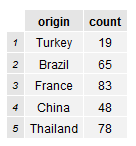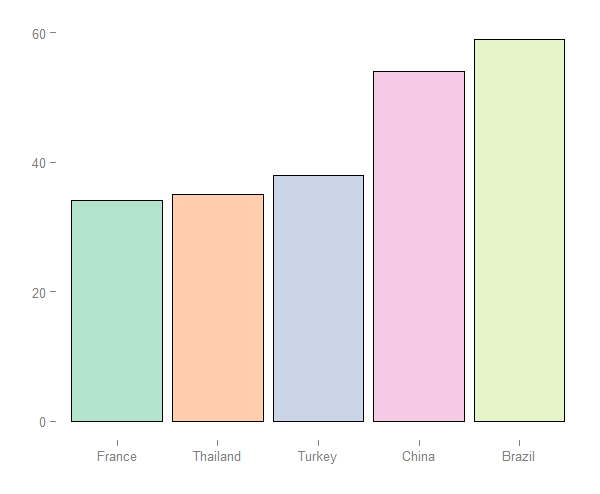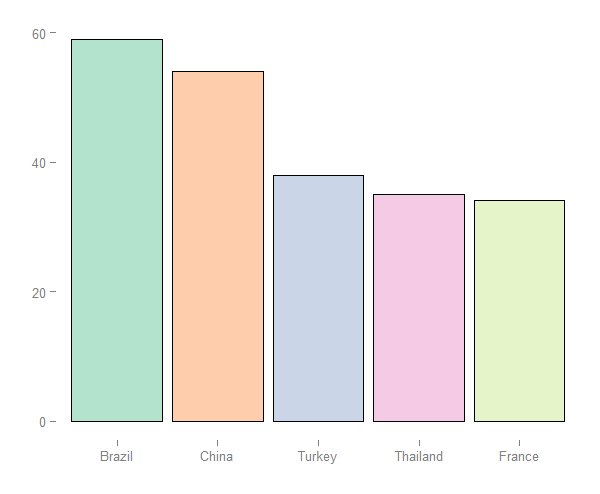Plot a clear ggplot2 bar chart with x-axis labels appearing in the frequency with which they occur in the data frame.

### Data Sample

Suppose we have a sample of students who come from different countries. This gives us a data frame of student origins, and the number of students who come from each origin.

``````#sample of student origin in class
cc.df````````````#plot is hard to interpret when origin ordered alphabetically
library(ggplot2)
country.count.plot <-
ggplot(cc.df, aes(x=origin, y=count)) +
geom_bar(stat="identity", colour="black", fill="white") +
xlab("") + ylab("")

country.count.plot``````### Solution (Up)

Using the reorder function we are able to order origin factor by ascending count.

``````#reorder origin by ascending count
cc.df\$origin <- reorder(cc.df\$origin, cc.df\$count)

#plot is much more clear
country.count.plot <-
ggplot(cc.df, aes(x=origin, y=count)) +
geom_bar(stat="identity", colour="black", fill="white") +
xlab("") + ylab("")

country.count.plot``````### Solution (Down)

We can also order origin by descending count.

``````#order by descending count
cc.df\$origin <- reorder(cc.df\$origin, -cc.df\$count)

#voila the plot I was looking for
country.count.plot <-
ggplot(cc.df, aes(x=origin, y=count)) +
geom_bar(stat="identity", colour="black", fill="white") +
xlab("") + ylab("")

country.count.plot``````### Feedback

Always feel free to get in touch with other solutions, general thoughts or questions.